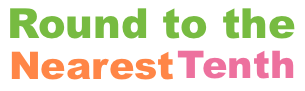Round 13 to the Nearest TenthThis is how to round 13 to the nearest tenth.

First note that 13 can also be written as 13.0, and 13.0 has two parts. The integer part to the left of the decimal point and the fractional part to the right of the decimal point:

Integer Part: 13
Fractional Part: 0

To round 13 (or 13.0) to nearest tenth means to round the numbers so you only have one digit in the fractional part.

13 (or 13.0) already has only one digit in the fractional part. Thus, 13 is already rounded as much as possible to the nearest tenth and the answer is:

13.0

Round To The Nearest Tenth
Here you can enter another number for us to round to the nearest tenth:

Round 13.01 to the Nearest Tenth
Here is the next number on our list that we rounded to the nearest tenth.

Copyright  |   Privacy Policy  |   Disclaimer  |   Contact